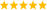# LabVIEW

cancel
Showing results for
Did you mean:

Solved!
Go to solution
Highlighted

## Converting 12 bit signed binary string to decimal

I am creating a new automated test program and could use some help with binary to decimal conversion. My program receives 32 bit binary strings, and I need to get the information stored in bits 18-29. I am using the "String Subset" function to extract the 12 bits I need but now I'm stuck on how to interpret them. The 12 bits should be interpreted as a signed binary number where the LSB=0.015625. If anyone can give me some advice I'd greatly appreciate it. Thank you.

Message 1 of 7
(419 Views)
Highlighted

## Re: Converting 12 bit signed binary string to decimal

Attach a simple VI containing a typical string.

Strings are quantized to bytes and you cannot get 12 bits as a subset. You can cast the string to a U32 and do some masking, shifting, etc to get your value. How are negative values encoded (two's complement, etc)LabVIEW Champion. It all comes together inGCentralWhat does "Engineering Redefined" mean??
Message 2 of 7
(393 Views)
Highlighted

## Re: Converting 12 bit signed binary string to decimal

Here are some typical binary strings and the correct interpretations of bits 18-29. It is signed as two's complement.

01100100100001110010000101101010  = 9.05

01100010110100010010000101101010 = 5.63

11111100011000010010000101101010= -7.24

01111110010000110010000101101010 = -3.47

Using the substring function I have been able to extract the 12 bits of interest, but I am stuck on translating those 12 bits to a decimal number.

Message 3 of 7
(374 Views)
Highlighted

## Re: Converting 12 bit signed binary string to decimal

Hi jflow,

get a subset of 16 digits, convert to I16, AND with xFFF0, divide to correct for your LSB resolution...

Best regards,
GerdWusing LV2011SP1 + LV2017 (+LV2020 sometimes) on Win10+cRIO
Message 4 of 7
(359 Views)
Highlighted
Solution
Accepted by jflow

## Re: Converting 12 bit signed binary string to decimal

@jflow wrote:

Here are some typical binary strings and the correct interpretations of bits 18-29. It is signed as two's complement.

01100100100001110010000101101010  = 9.05

01100010110100010010000101101010 = 5.63

11111100011000010010000101101010= -7.24

01111110010000110010000101101010 = -3.47

Using the substring function I have been able to extract the 12 bits of interest, but I am stuck on translating those 12 bits to a decimal number.

Are these strings 32 characters consisting of either "0" or "1"?  Of do you actually have 4 characters and you are just showing the values in a binary format?  It does actually make a difference.  This is why it is advised to supply an example VI with the values saved as default so we know exactly what you are dealing with.

So assuming you mean literal string of "0" or "1", I would do the followingThere are only two ways to tell somebody thanks: Kudos and Marked Solutions
Unofficial Forum Rules and Guidelines
Message 5 of 7
(265 Views)
Highlighted

## Re: Converting 12 bit signed binary string to decimal

@crossrulz wrote:
Are these strings 32 characters consisting of either "0" or "1"?

That's why I was asking for some code. Originally, it was described as "32bit binary string" while your interpretation would be 32 bits formatted in binary, i.e. a string with 32 characters, i.e. 256 bits total.

The term "binary string" is typically reserved for strings that can contain any possible bit pattern, even non-readable. A "formatted string" of zeroes and Ones can only contain two possible characters and is thus an eight-fold waste of bits. What kind of device would produce something like that??? 😄

(From the original question, there was no way to tell that this will actually be the solution ;))LabVIEW Champion. It all comes together inGCentralWhat does "Engineering Redefined" mean??
Message 6 of 7
(230 Views)
Highlighted

## Re: Converting 12 bit signed binary string to decimal

@altenbach wrote:

@crossrulz wrote:
Are these strings 32 characters consisting of either "0" or "1"?

That's why I was asking for some code. Originally, it was described as "32bit binary string" while your interpretation would be 32 bits formatted in binary, i.e. a string with 32 characters, i.e. 256 bits total.

And later in that paragraph, I reemphasized this point.

I only figured the OP had this horrible format since they mentioned using String Subset.

@altenbach wrote:

The term "binary string" is typically reserved for strings that can contain any possible bit pattern, even non-readable. A "formatted string" of zeroes and Ones can only contain two possible characters and is thus an eight-fold waste of bits. What kind of device would produce something like that??? 😄

Well, don't go digging into NI's RF Modulation Toolkit then.There are only two ways to tell somebody thanks: Kudos and Marked Solutions
Unofficial Forum Rules and Guidelines
Message 7 of 7
(225 Views)